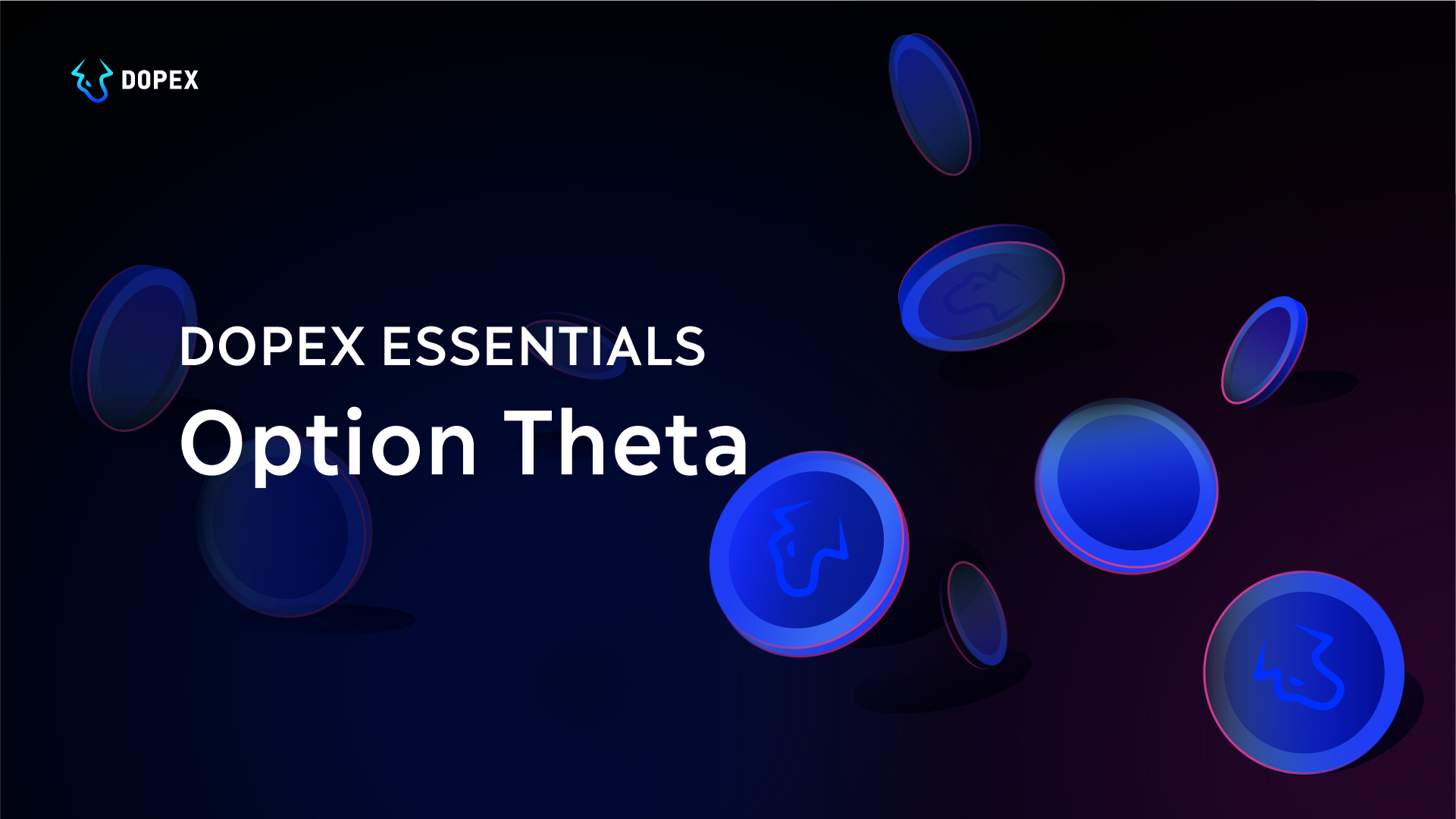# Dopex Essentials: Option Theta

Dopex-Essentials# The Silverback Odyssey

## Here is what may be going through his mind:

• He knows he carries unlimited risk and limited reward potential
• He also knows that by virtue of time, there is a chance for the option he is selling to transition into the ITM option, which means he will not get to retain the premium received for writing the option

## When you pay a premium for options, you are paying towards:

1. Time Induced Risk
2. The option’s intrinsic value

## Example

###### Assuming that Pump Token is trading at $1,423 — let us calculate the value of the following options: 1.$1,350 Call Option
2. $1,450 Call Option 3.$1,400 Put Option
4. $1,450 Put Option ## First, remember these points 1. The intrinsic value can never go below 0. If the value is negative then the intrinsic value is considered to be zero. 2. Intrinsic value for Call options is calculated in the following way “Spot Price — Strike Price 3. Intrinsic value for Put options is calculated in the following way “Strike Price — Spot Price ## With that in mind, we can calculate the intrinsic values of the aforementioned options 1.$1,350 Call Option = $1,423 —$1,350 = +73
2. $1,450 Call Option =$1,423 — $1,450 = 0 (negative value) 3.$1,400 Put Option = $1,400 —$1,423 = 0 (negative value)

## Let us take another example

• Underlying Asset Price = $1,514 • Strike Price =$1,450
• Option Type: Call Option
• Option Moneyness = ITM
• Date of purchase = 6th July 2021
• Date of Expiry = 30th July 2021

# Glossary of terms

###### In the money (ITM):
• For a call — this term is used when the strike price is lower than the current price of the underlying asset.
• For a put — this term is used when the strike is higher than the current price.
###### At the money (ATM):
• For both a call and a put — this term is used when the strike is equal to the current price.
###### Out of the money (OTM):
• For a call — this term is used when the strike price is higher than the current price of the underlying asset.
• For a put — this term is used when the strike is lower than the current price.

← Back to Blog